# Find the minimum and maximum values of the function (x, y, z) = x + y...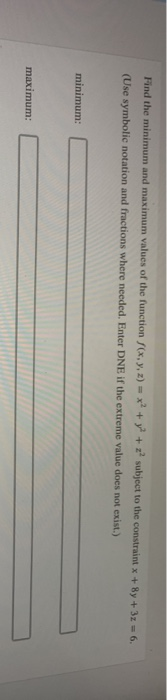Find the minimum and maximum values of the function (x, y, z) = x + y + z subject to the constraint x + 8y + 32 = 6. (Use symbolic notation and fractions where needed. Enter DNE if the extreme value does not exist.) minimum: maximum: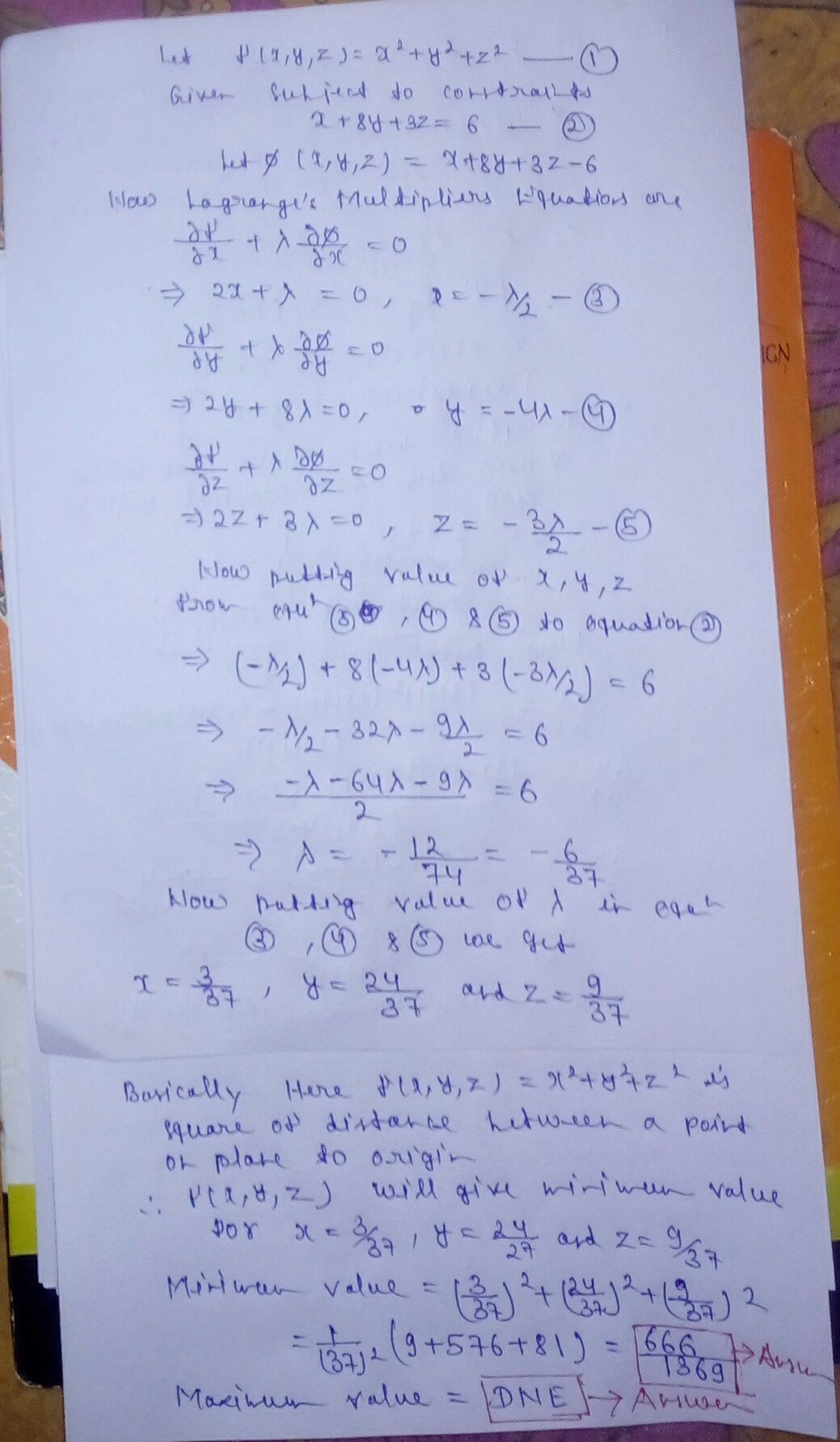#### Earn Coin

Coins can be redeemed for fabulous gifts.

Similar Homework Help Questions
• ### 3. Find the minimum and maximum values of the function f (x, y) = x2 + y subject to the constraint x y = 162. Use the L...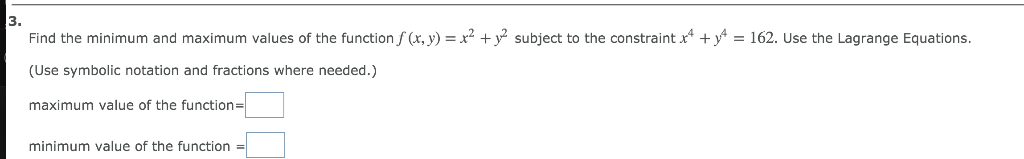3. Find the minimum and maximum values of the function f (x, y) = x2 + y subject to the constraint x y = 162. Use the Lagrange Equations. (Use symbolic notation and fractions where needed.) maximum value of the function| minimum value of the function 3. Find the minimum and maximum values of the function f (x, y) = x2 + y subject to the constraint x y = 162. Use the Lagrange Equations. (Use symbolic notation and fractions...

• ### 3 Find the minimum and maximum values of the function f(x, y)= x +y subject to the constraint x + y 1250. Use the L...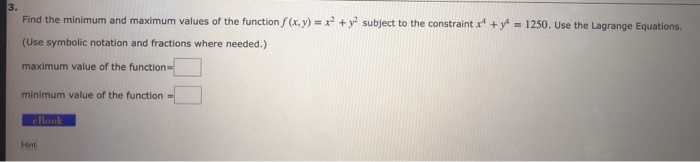3 Find the minimum and maximum values of the function f(x, y)= x +y subject to the constraint x + y 1250. Use the Lagrange Equations. (Use symbolic notation and fractions where needed.) maximum value of the function minimum value of the function cBook Hint 3 Find the minimum and maximum values of the function f(x, y)= x +y subject to the constraint x + y 1250. Use the Lagrange Equations. (Use symbolic notation and fractions where needed.) maximum value...

• ### Find the extreme values of the function f(x, y) = 3x + 6y subject to the...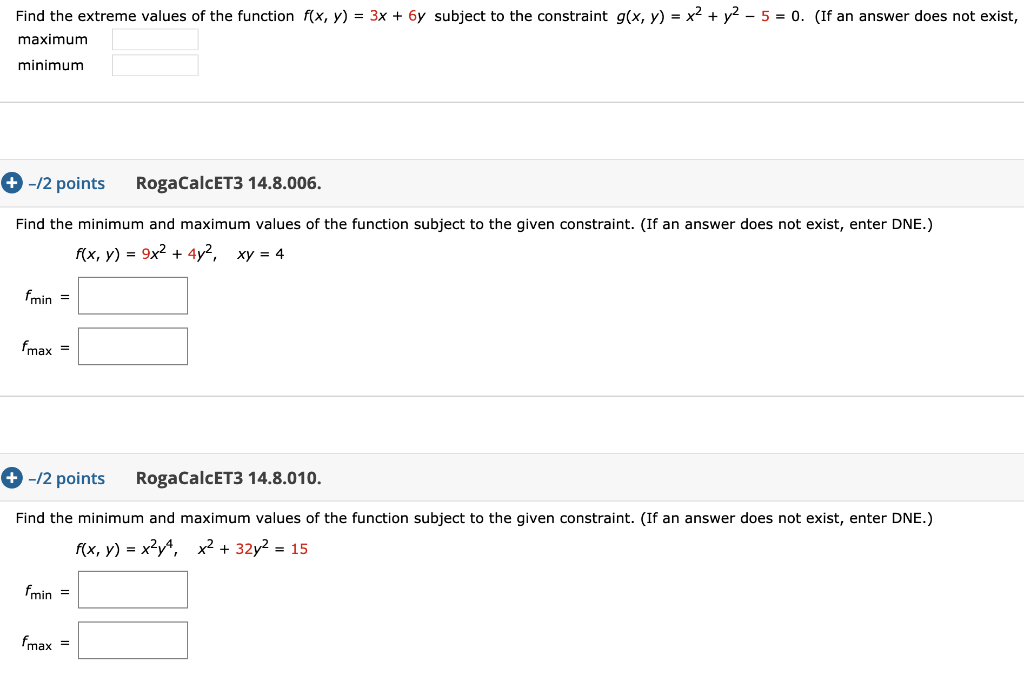Find the extreme values of the function f(x, y) = 3x + 6y subject to the constraint g(x, y) = x2 + y2 - 5 = 0. (If an answer does not exist, maximum minimum + -/2 points RogaCalcET3 14.8.006. Find the minimum and maximum values of the function subject to the given constraint. (If an answer does not exist, enter DNE.) f(x, y) = 9x2 + 4y2, xy = 4 fmin = Fmax = +-12 points RogaCalcET3 14.8.010. Find...

• ### Use Lagrange multipliers to find the maximum and minimum values of the function subject to the given constraint. (If a v...

Use Lagrange multipliers to find the maximum and minimum values of the function subject to the given constraint. (If a value does not exist, enter NONE.) f(x,y,z) = x2 + y2 + z2;   x4 + y4 + z4 = 1

• ### + ww. Consider the function below. x)e-1/x+6) (a) Find the vertical asymptote(s). (Enter your answers as...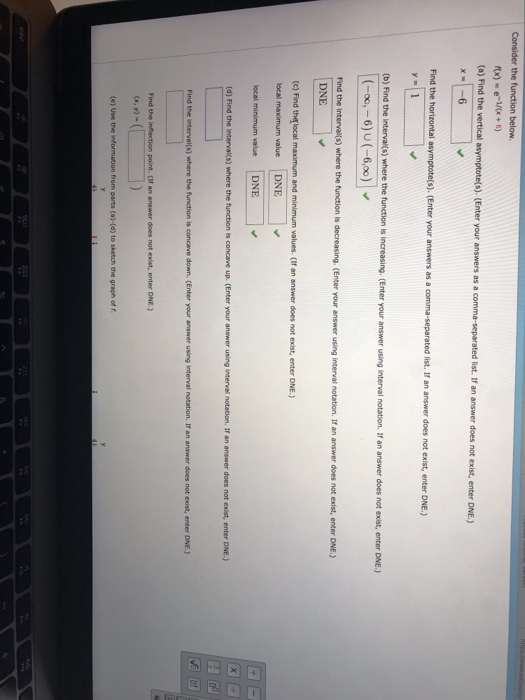+ ww. Consider the function below. x)e-1/x+6) (a) Find the vertical asymptote(s). (Enter your answers as a comma-separated list. If an answer does not exist, enter DNE.) x -6 Find the horizontal asymptote(s). (Enter your answers as a comma-separated list. If an answer does not exist, enter DNE.) y 1 (b) Find the interval(s) where the function is increasing. (Enter your answer using interval notation. If an answer does not exist, enter DNE.) (-o0, - 6) U (-6,00) Find the...

• ### Consider the function below. x)= (x-4)2 (a) Find the vertical asymptote(s). (Enter your answers as a...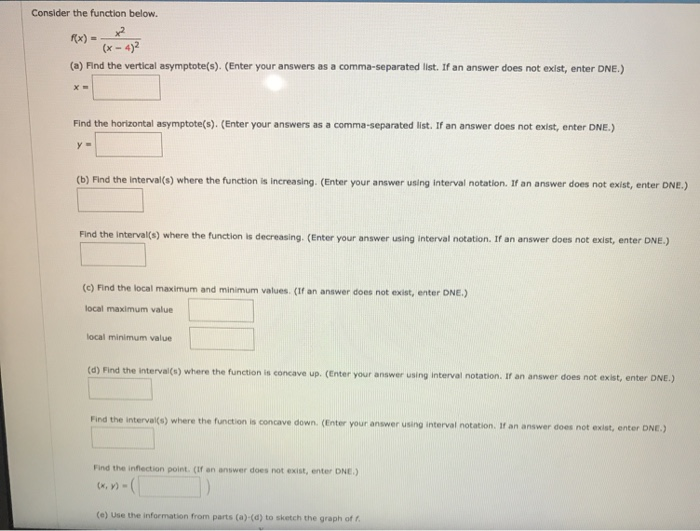Consider the function below. x)= (x-4)2 (a) Find the vertical asymptote(s). (Enter your answers as a comma-separated list. If an a er does not exist, enter DNE.) answ x Find the horizontal asymptote(s). (Enter your an comma-separated list. If an answer does not exist, enter DNE.) s wers as a y (b) Find the interval(s) where the function is increasing. (Enter your answer using interval notation. If an answer does not exist, enter DNE.) Find the interval(s) where the function...

• ### This extreme value problem has a solution with both a maximum value and a minimum value....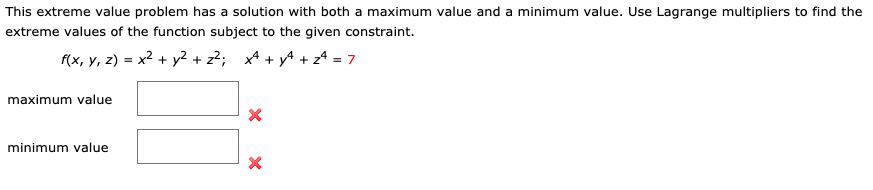This extreme value problem has a solution with both a maximum value and a minimum value. Use Lagrange multipliers to find the extreme values of the function subject to the given constraint. f(x, y, z) = x2 + y2 + z2;    x4 + y4 + z4 = 7 Maximum Value: Minimum Value: This extreme value problem has a solution with both a maximum value and a minimum value. Use Lagrange multipliers to find the extreme values of the function subject to...

• ### 11 Find any I the extreme values (if of the given function f(x, y, 2) =...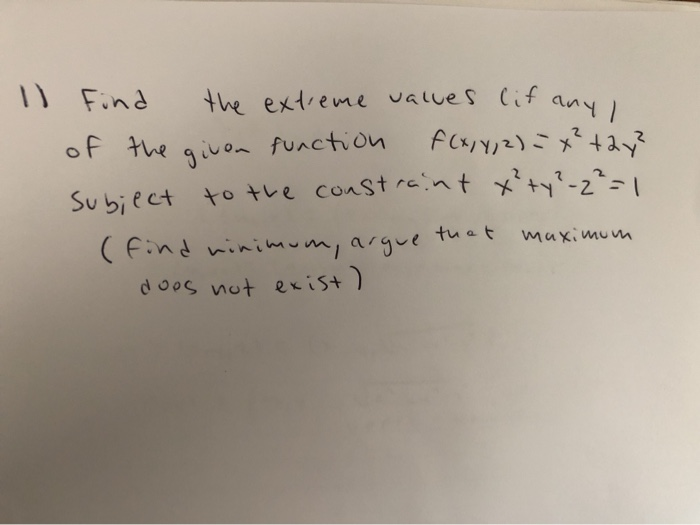11 Find any I the extreme values (if of the given function f(x, y, 2) = x² + 2y? subject to the constraint x²+y²-2²=1 (find minimum, argue that does not exist ) maximum

• ### The function f(x,y,z) = 7x has an absolute maximum value and absolute minimum value subject to...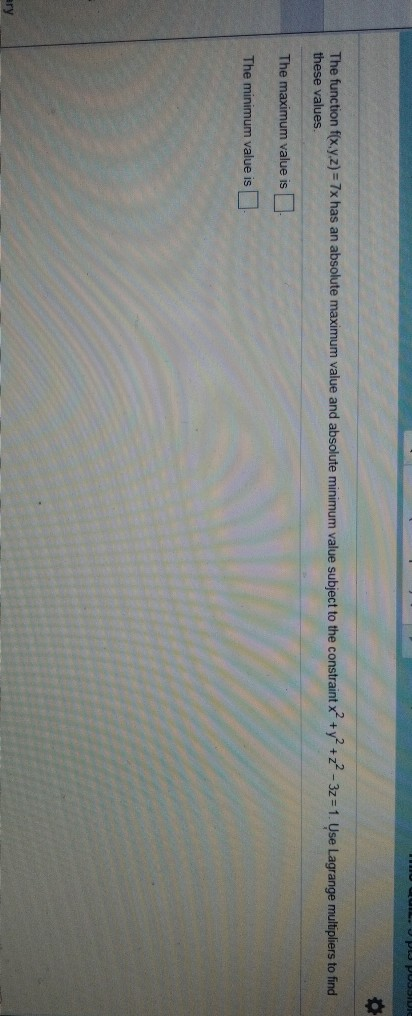The function f(x,y,z) = 7x has an absolute maximum value and absolute minimum value subject to the constraint x +y +z - 3z = 1. Use Lagrange multipliers to find these values. The maximum value is - The minimum value is

• ### Find the maximum and minimum values of the function and the values of x and y...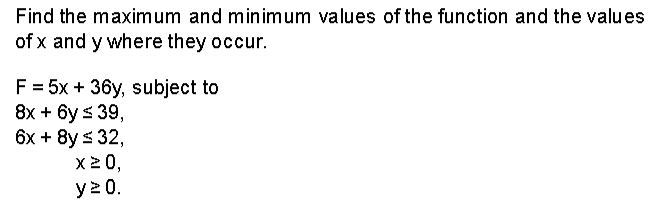Find the maximum and minimum values of the function and the values of x and y where they occur. F = 5x + 36y, subject to 8x + y s 39, 6x + y 32, x20, y20.